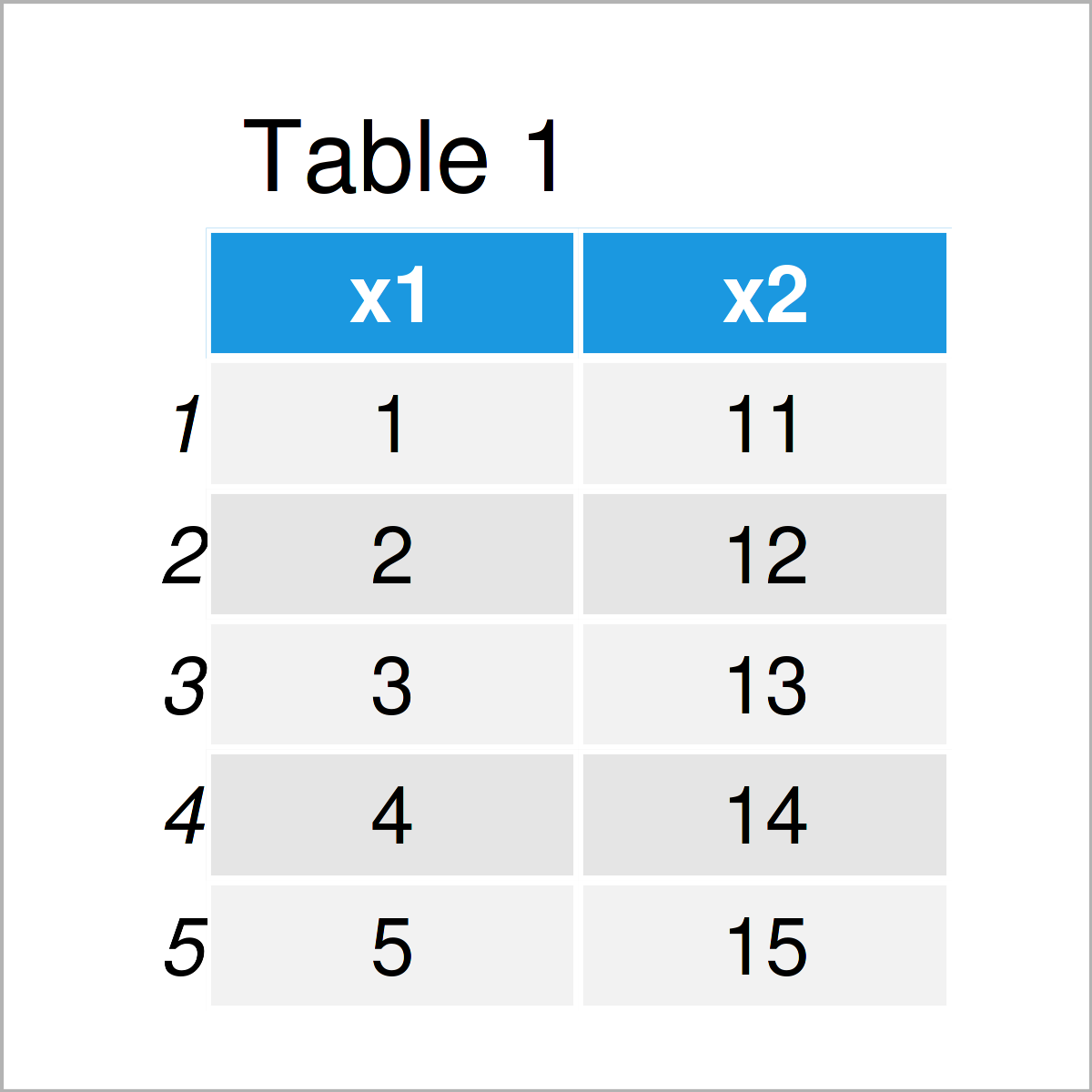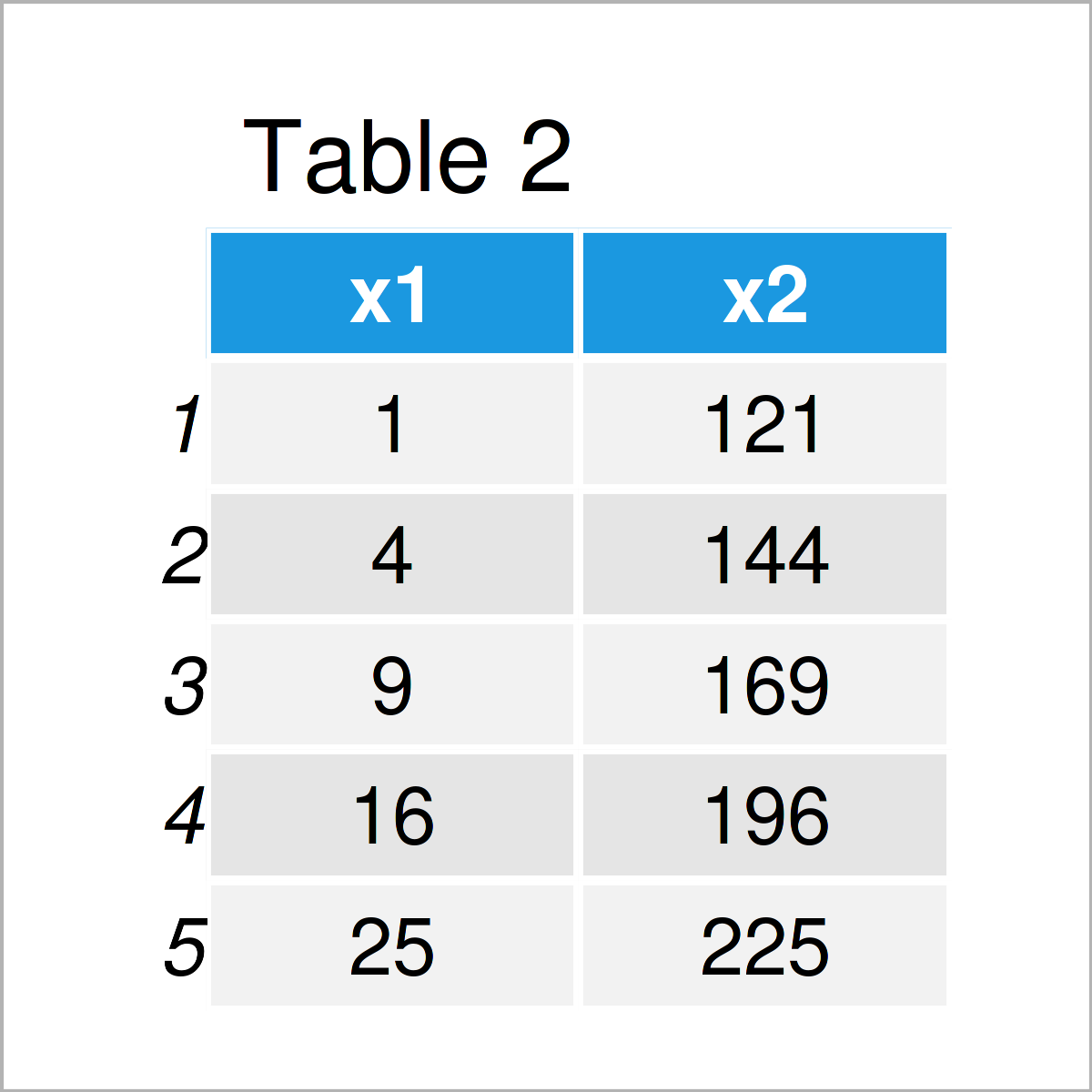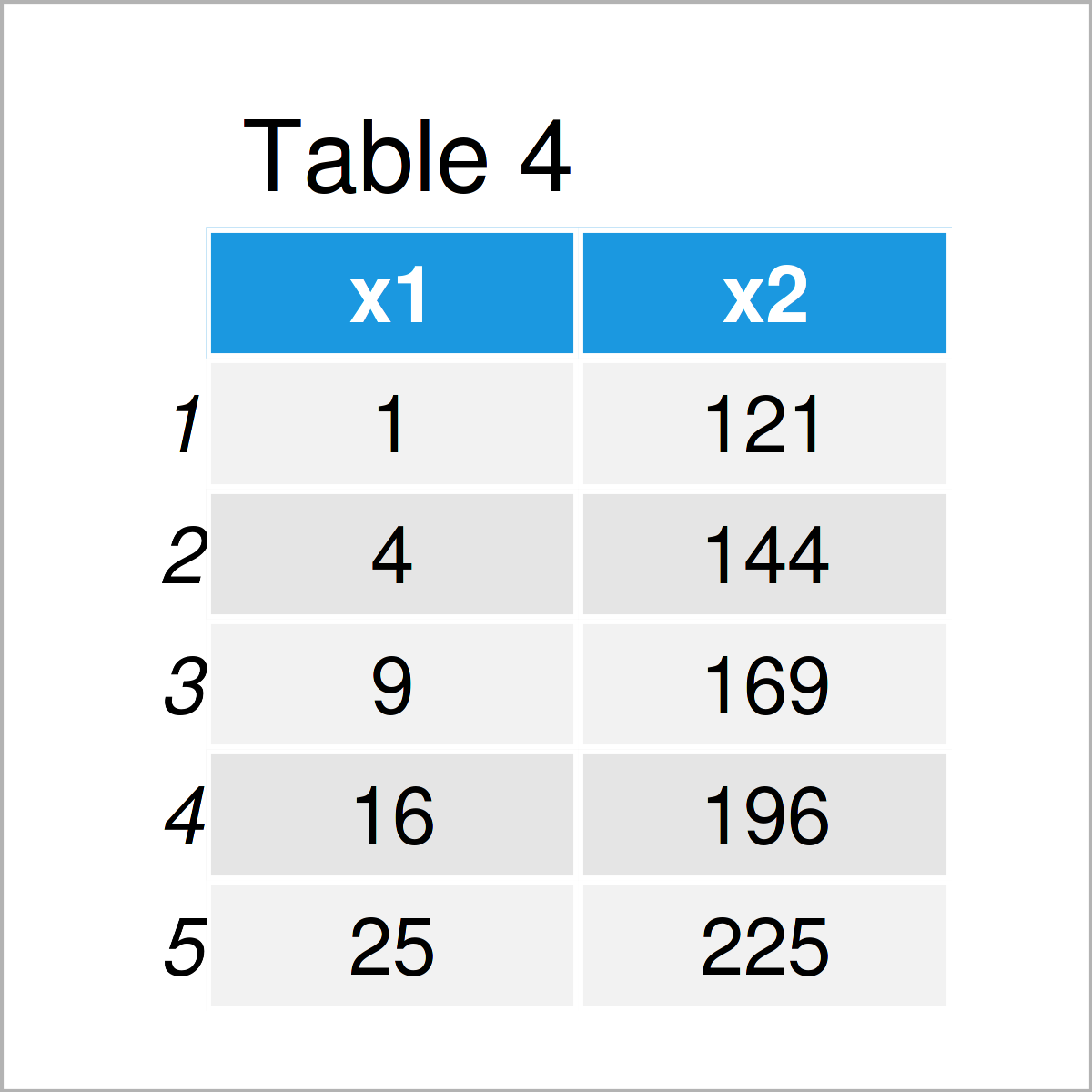# Update Data Frame with Function in R (Example)

In this tutorial you’ll learn how to update a data frame with a user-defined function in R programming.

The tutorial will contain the following topics:

Here’s how to do it.

## Creation of Example Data

Initially, we’ll need to create some data that we can use in the following examples:

```my_data <- data.frame(x1 = 1:5, # Create example data x2 = 11:15) my_data # Print example data```Have a look at the table that got returned after running the previous R code. It shows that our example data consists of five rows and two variables.

Next, we have to create a user-defined function that should be used to update our data frame:

```my_fun <- function(my_data) { # Create user-defined function my_data <- my_data^2 my_data }```

So far, so good! Let’s update our data!

## Example: Update Data Frame via Function

In this example, I’ll illustrate how to update a data frame using a user-defined function in R.

Let’s apply our function to our data frame:

`my_fun(my_data) # Apply user-defined function`By executing the previous code, we have constructed Table 2, i.e. an updated version of our data frame.

Looks good, doesn’t it?

However, if we print our data frame once again without wrapping the function around it, the original input data is returned:

`my_data # Updating data frame via function doesn't work`The reason for this is that our function has a different environment inside and outside the function.

For that reason, we have to store the output of our function in a new data object (in this case, we are just updating the content of the input data):

```my_data <- my_fun(my_data) # Properly store output of function my_data # Print updated data frame```As shown in Table 4, the previously shown R code has created an updated version of our data frame.

## Video & Further Resources

Have a look at the following video on my YouTube channel. I’m explaining the R codes of this tutorial in the video.

In addition to the video, you may want to have a look at the related tutorials on my homepage. You can find some other articles below.

To summarize: In this R programming tutorial you have learned how to modify a data frame with a function call. Let me know in the comments section, if you have any further questions.

Subscribe to the Statistics Globe Newsletter